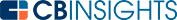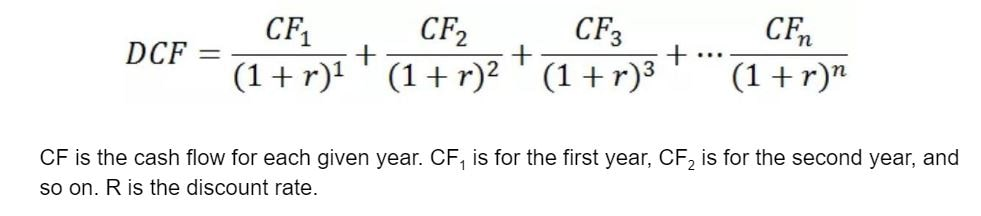# What is Discounted Cash Flow?Discounted cash flow (DCF) is a valuation method that estimates the value of an investment based on its expected future cash flows.

DCF analysis aims to estimate how much an investor will receive from a long-term investment. It takes into account the time value of money. This is the concept that money is more valuable in the present than in the future due to its ability to earn compound interest.

## How is discounted cash flow calculated?

Discounted cash flow is calculated using the following formula:The discount rate is normally the weighted average cost of capital (WACC). This incorporates the annual rate of return that shareholders expect that year. The WACC is essentially the opportunity cost of spending money on the investment rather than on alternative investments.

## What are the advantages of discounted cash flow?

Using DCF allows companies to evaluate investment opportunities. It has a number of advantages, such as:

• It’s suitable for analyzing a wide range of potential investments, including mergers and acquisitions.
• It’s a detailed method that includes all major assumptions and future expectations about a business. Investors can incorporate key changes in the business strategy (other valuation models, such as relative and APV, will not reflect these).
• It’s a straightforward formula, meaning that the DCF analysis can be performed in Excel or another spreadsheet tool.

## What are the disadvantages of discounted cash flow?

Some of the key problems with DCF are that:

• The cash flow projection for each year in the model may be uncertain, with this uncertainty increasing with each additional year. Discounted cash flow models often use 5 or even 10 years of estimates. Small errors or incorrect assumptions in the early years can have a huge effect on the later years.
• Capital expenditure assumptions can be inaccurate. Again, the potential for inaccuracies grows with each year in the model.
• The discount rate and growth rate assumptions may not be correct. Using the weighted average cost of capital (WACC) is common, but this may not work well for real-world (rather than theoretical) investments.

Discounted cash flow is a valuable formula investors can use to evaluate a potential investment based on the estimated future returns. It’s not the only way to assess potential investment opportunities, but when used with other formulas, it can help predict an investment’s profitability potential.

You may also like# www.cbinsights.com